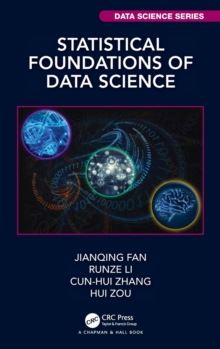# Statistical Foundations of Data Science Hardback

## Part of the Chapman & Hall/CRC Data Science Series series

Hardback

• Information

#### Description

Statistical Foundations of Data Science gives a thorough introduction to commonly used statistical models, contemporary statistical machine learning techniques and algorithms, along with their mathematical insights and statistical theories.

It aims to serve as a graduate-level textbook and a research monograph on high-dimensional statistics, sparsity and covariance learning, machine learning, and statistical inference.

It includes ample exercises that involve both theoretical studies as well as empirical applications. The book begins with an introduction to the stylized features of big data and their impacts on statistical analysis.

It then introduces multiple linear regression and expands the techniques of model building via nonparametric regression and kernel tricks.

It provides a comprehensive account on sparsity explorations and model selections for multiple regression, generalized linear models, quantile regression, robust regression, hazards regression, among others.

High-dimensional inference is also thoroughly addressed and so is feature screening.

The book also provides a comprehensive account on high-dimensional covariance estimation, learning latent factors and hidden structures, as well as their applications to statistical estimation, inference, prediction and machine learning problems.

It also introduces thoroughly statistical machine learning theory and methods for classification, clustering, and prediction.

These include CART, random forests, boosting, support vector machines, clustering algorithms, sparse PCA, and deep learning.

#### Information

• Format:Hardback
• Pages:752 pages, 100 Illustrations, black and white
• Publisher:Taylor & Francis Inc
• Publication Date:
• Category:
• ISBN:9781466510845

£99.99

£81.06

£74.99

£61.88

£68.99

£57.28

£42.99

£37.29

£105.00

£84.90